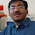### Coin Toss Bankruptcy

Source: Mailed to me by Sudeep Kamath (EECS PhD Student, UC Berkeley, EE IITB 2008 Alumnus)

Problem: Three people start with integer amounts a,b and c. In each round, each one tosses a fair coin. If not all faces are the same, the person with the different face gets a rupee from each of the other two. If all faces are the same, no money is exchanged. This process is repeated till one of them gets bankrupt. What is the expected number of rounds till the game ends?

Related Problems:
http://pratikpoddarcse.blogspot.com/2009/10/lets-say-keep-tossing-fair-coin-until.html
http://pratikpoddarcse.blogspot.com/2010/11/source-credit-suisse-placement-test-at.html

Update (15/03/2011):
Hint: Given away by Sudeep. (* Define a martingale of the form
Y_n=A_n*B_n*C_n + some other term (where A_n,B_n,C_n are the fortunes
of the three players at time n). *)
Solution: Posted by chera (Gaurav Sinha, IITK 1996 Graduate, Indian Revenue Service) in comments!

1.I'm not sure... But is it fair to say that the game is expected to continue forever because in each turn the expected return for each person is 0?

2.Can you give a more mathematical argument?

3.His Hint:

Define a martingale of the form
Y_n=A_n*B_n*C_n + some other term (where A_n,B_n,C_n are the fortunes
of the three players at time n).

Then, define the time when the product A_n*B_n*C_n first becomes 0 as a stopping time and use the Optional Stopping Theorem.

4.let n denotes the number of rounds completed. suppose (a_n,b_n,c_n) are fortunes after n rounds.

define a sequence of r.v.
Y(n)= abc+(n)*(3/4)*[a+b+c-2].
where a=a_n, b= b_n, c=c_n.

after (n+1)th round, there are 4 possible states {(a,b,c),(a+2,b-1,c-1),(a-1,b+2,c-1),(a-1,b-1,c+2) all with probability 1/4.

it is straightword calculation to see that given result of game after n rounds, E Y(n+1)= Y(n). thus sequence of r.v.s Y(n) is martingale.

if T is the stopping time, i.e. after T rounds, the game is over then,
E Y[T] = 3/4 * E [T] *(a+b+c-2)
Y = abc, where a,b,c are fortunes at beginning.

as per optimal stopping theorem
E Y[T] = Y .

so we get E[T]= (4/3)*abc/(a+b+c-2)

5.Wow.. Quite an interesting problem.. was trying to come up with a martingale for a while.. saw the hint finally...
M_n = an*bn*cn+n*(an+bn+cn) - 2n is a martingale.. hence
expected number of rounds is just half the product of the initial fortunes divided the sum less 2.

6.@Siva. Please check again. I do not think your random variable is a martingale.

7.OOps... I was solving a problem where money is exchange at every round.. hence I missed the 4/3 factor..

8.Just to be mathematically precise.. your claim
E Y(n+1)= Y(n) can be written more precisely as
E [Y(n+1) | Filtration(n)] = Y(n)

Rest follows. Thanks a ton.